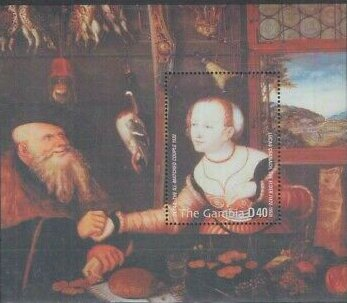## Image Colnect-6016-193-The-ill-matched-couple.jpgNo higher resolution available (347 x 303 pixels) Type: sheet Issuer: Gambia Date: 2003 Visibility: public Image owner: none 116 matches in all, showing first 100: empty sheet (#13846) empty sheet (#53480) empty sheet (#53481) empty sheet (#53482) empty sheet (#53483) empty sheet (#53484) empty sheet (#53485) empty sheet (#53486) empty sheet (#53487) empty sheet (#53488) empty sheet (#53489) empty sheet (#53490) empty sheet (#53491) empty sheet (#53492) empty sheet (#53493) empty sheet (#53494) empty sheet (#53495) empty sheet (#53496) empty sheet (#53497) empty sheet (#53498) empty sheet (#53499) empty sheet (#53500) empty sheet (#53501) empty sheet (#53502) empty sheet (#53503) empty sheet (#53504) empty sheet (#53505) empty sheet (#53506) empty sheet (#53507) empty sheet (#53508) empty sheet (#53509) empty sheet (#53510) empty sheet (#53511) empty sheet (#53512) empty sheet (#53513) empty sheet (#53514) empty sheet (#53515) empty sheet (#53516) empty sheet (#53517) empty sheet (#53518) empty sheet (#53519) empty sheet (#53520) empty sheet (#53521) empty sheet (#53522) empty sheet (#53523) empty sheet (#53524) empty sheet (#53525) empty sheet (#53526) empty sheet (#53527) empty sheet (#53528) empty sheet (#53529) empty sheet (#53530) empty sheet (#53531) empty sheet (#53532) empty sheet (#53533) empty sheet (#53534) empty sheet (#53535) empty sheet (#53536) empty sheet (#53537) empty sheet (#53538) empty sheet (#53539) empty sheet (#53540) empty sheet (#53541) empty sheet (#53542) empty sheet (#60820) empty sheet (#60821) empty sheet (#60822) empty sheet (#60823) empty sheet (#60824) empty sheet (#60825) empty sheet (#60826) empty sheet (#60827) empty sheet (#60828) empty sheet (#60829) empty sheet (#60830) empty sheet (#60831) empty sheet (#60832) empty sheet (#60833) empty sheet (#60834) empty sheet (#60835) empty sheet (#60836) empty sheet (#60837) empty sheet (#60838) empty sheet (#60839) empty sheet (#60840) empty sheet (#60841) empty sheet (#60842) empty sheet (#60843) empty sheet (#60844) empty sheet (#60845) empty sheet (#60846) empty sheet (#60847) empty sheet (#60848) empty sheet (#60849) empty sheet (#60850) empty sheet (#60851) empty sheet (#60852) empty sheet (#60853) empty sheet (#60854) empty sheet (#60855) Crops No visible images cropped from this one. Measurements Resolution is unknown. Source Source name: Colnect File URL: http://i.colnect.net/b/6016/193/The-ill-matched-couple.jpg Uploaded December 2, 2019, 8:40 pm by Stan Shebs